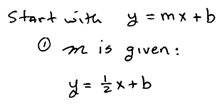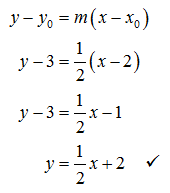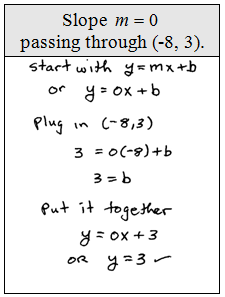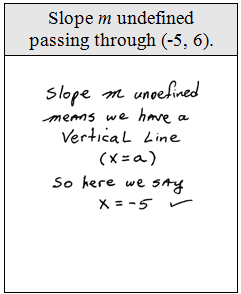## Pages

### Finding Linear Equations

Slope intercept form also allows us to easily find the equation of the line given the graph.  If you are given the slope and any point on the line you can find its equation by following the steps below:

Example: Find the equation of the line passing through (2, 3) with slope m = 1/2.

Step 1: Use y = mx + b and find m.Step 2: Use the given point to find b.Step 3: Put it all together using y = mx + b.Alternatively, you can use point-slope form to answer the same question.Substitute (2, 3) and slope m = 1/2  into the formula as follows:Either method is valid and the answer is the equation.

Find the equation of the given line.